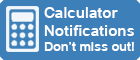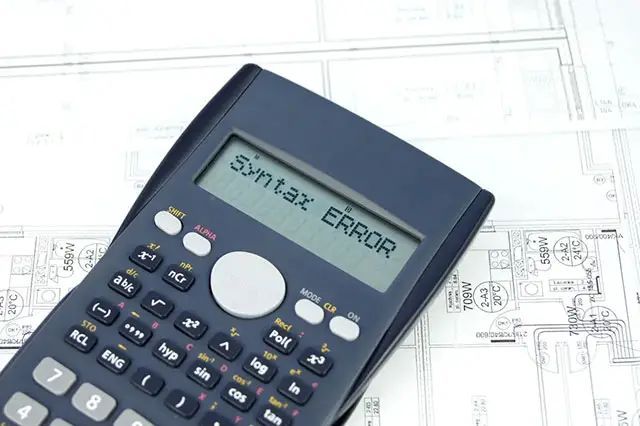Calculator Library
Free Online CalculatorsHome » 87 Calculators » Blog » Common Calculator Mistakes

## COMMON CALCULATOR MISTAKESThere are many potential mistakes a person can make when using a calculator. Some are input errors, some are syntax errors, others are calculation errors, and many are caused by the calculator using a different logic process or working to a specific set of rules that the user is unfamiliar with.

Often a calculator will throw an error message when a mistake is made, but sometimes it will simply give you an incorrect result. This is where it's a good idea to use common sense and ask yourself, "does that answer look right?". Don't always believe every answer your calculator gives you.

Let's start with the most fundamental mistake and move on from there.

## Failing To Clear Previous Calculations

As you work through different problems on your calculator, one of the easiest mistakes is to forget to clear your existing data from previous calculations before starting new ones. The process varies on different calculators, however you need to clear everything from the display, memory, registers or the stack. The AC key will do this on many calculators, however some calculators will have a special key combination to clear memory. For example, the TI-84 Plus uses the key combination 2nd + 7 1 2 clear. Alternatively, many financial calculators use the key combination f CLx.

## Wrong Key For Negative Numbers

Calculator users sometimes mistake the subtraction key - for the negative or change sign key, either (-) or +/-. If you use the subtraction key at the beginning of a calculation, the calculator thinks you want to subtract a value from the previous answer. Alternatively, using the subtraction key to set a negative number during a calculation can result in an error message.

## Wrong Base For Logarithms

Most calculators can do both base 10 and base "e" logarithms, but it's easy to confuse logarithm keys. Remember to use the log key for base 10 logarithms and the ln key for base "e" logarithms.

## Using Radian Mode To Enter Angles In Degrees

When performing trigonometric calculations, angles can be entered in one of two units of measurement, either Radians or Degrees. Many calculators use Radians as the default for these calculations, so you'll get the wrong answer if you're working in Degrees. In this scenario, you must switch the calculator to Degrees before calculating your result.

## Deactivated Functions

Many calculators allow functions to be turned off and on. Usually, a function is turned on when the equals sign in its location is highlighted, so you'll have problems if you want to use a function where the equals sign is not highlighted. To fix the problem, simply move the cursor to the relevant equals sign and press the ENTER key.

## Rounding Errors

Numbers are commonly rounded up or down to fit within a fixed or maximum number of decimal places. The general rule is that numbers 1-4 are rounded down while numbers 5-9 are rounded up. An obvious example of rounding is currency, where a calculation that results in an exact value of 10.123 will be rounded down to \$10.12 due to the currency having two fixed decimal places.

A rounding error, or roundoff error, is the difference between a number's close approximation and its exact value. Rounding errors can be demonstrated easily using percentages, as shown below.

 First, take a list of exact percentages... ...then round to the nearest whole number. 15.597% 16% 38.675% 39% 45.728% 46% TOTAL: 100.000% TOTAL: 101%

As you can see, rounding leads to an impossible result of 101%. In more complicated calculations, rounding errors can grow significantly as they are passed on through multiple calculation steps.

Calculators can only use a limited number of digits in their computations, so rounding occurs regularly. However, most calculators let you control where rounding happens by selecting the number of digits after the decimal point. Some calculators, such as the TI-84 Plus, also give you a "Float" option where the calculator decides how many decimals to use.

## Financial Calculator Mistakes

Several mistakes are typical when using the time value of money components on financial calculators. These include the following.

### Inconsistent Time Frequency Values

A significant mistake is entering inconsistent time frequency values. When performing time value of money calculations, it’s vital that the frequency of N (number of periods), I (interest rate) and PMT (payments per period) components must match. For example, if "N" is entered as a monthly value, the "I" and "PMT" values must also be expressed monthly.

### Wrong Payment Period Setting

Another potential mistake can be caused by using an incorrect payment period setting. Some payments, such as mortgages, are usually due at the end of a payment period, while others such as annuities are due at the beginning of a payment period. As a result, it's essential to ensure that the payment period on your calculator is set correctly for the calculation being performed.

If you're performing end of payment period calculations, check that the "BGN" icon isn't shown on the calculator's display. Most calculators default to "end of payment period" calculations and don't show this setting on the display. However, if the calculator is set to "beginning of payment period", a "BGN" icon will usually be displayed.

## Your Calculator's Logic May Be Different To Yours

Many calculator mistakes are caused by your calculator "thinking" differently than you do or following different rules. Here are some examples where this results in unexpected answers.

### Squaring Negative Numbers

If you try to calculate −32 using the button sequence 3 +/- x2 = you'll get the answer -9 instead of 9 as you may expect. Why does this happen?

The problem is caused by the way you're entering the data. The calculator reads your entry as −(32) = −9 because it performs exponentiation before negation. The solution is to put -3 in brackets. In other words, enter your data as (−3)2.

If you try to add 10% + 10% you'll get the answer 0.11 instead of 20% as you may expect. What's happening here, and how do you get the correct answer?

The calculator reads your entry as adding 0.1 times 10% to 10% (or 0.1); in other words, 0.1 + 0.1 x 10% = 0.11. The solution is to use one of the following options:

1. Convert the percentages to decimals, so the calculation becomes 0.1 + 0.1.
2. Add parentheses to the percentages, so the calculation becomes (10%) + (10%).
3. Add parentheses to the numbers and put the percentage sign outside them, so the calculation becomes (10 + 10)%.

 Previous Post« History Of The Calculator Next PostFinding Hidden Calculators »